Sunday 31st May 2020

CBSE Guess > Papers > Question Papers > Class XII > 2004 > Maths > Outside Delhi Set-II

MATHS (Set II—Outside Delhi)

Except for the following questions, all the remaining questions have been asked in Set I .

SECTION - A

Q. 6. Using properties of determinants, Prove that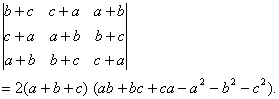Q. 7. Solve the differential equation: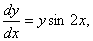Given that y (0) =1.

Q. 12. Prove that: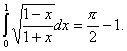Or

Prove that: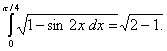Q. 13. If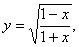Prove that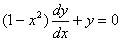Q. 17. Prove that the area of the region included between the parabola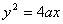and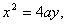where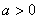is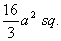SECTION - B

Q. 19. Show that the four points whose position vectors are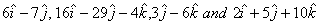are coplanar.

Q. 21. Find the equation of the plane passing through the point (-1, -1, 2) and perpendicular to the planes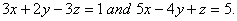Q. 24. Three forces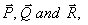acting on a particle, are in equilibrium. If the angle between forces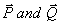is double the angle between the forces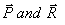show that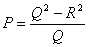SECTION - C

Q. 20. A is known to speak the truth 3 times out of 5 times. He throws a die and reports that it is 1. Find the probability it is actually 1.

Q. 21. If the banker's gain on bill due 4 months hence at 6% p.a. is Rs.400, find the amount of the bill and also find the true discount on it.

Q. 23. The cost function and revenue function of x units of an item are given by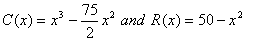respectively. Find the number of items to be produced and sold to have maximum profit, find the maximum profit also.

 Maths 2004 Question Papers Class XII Delhi Outside Delhi Compartment Delhi Compartment Outside DelhiSet ISet ISet ISet ISet IISet IISet IISet IISet IIISet III

CBSE 2004 Question Papers Class XII